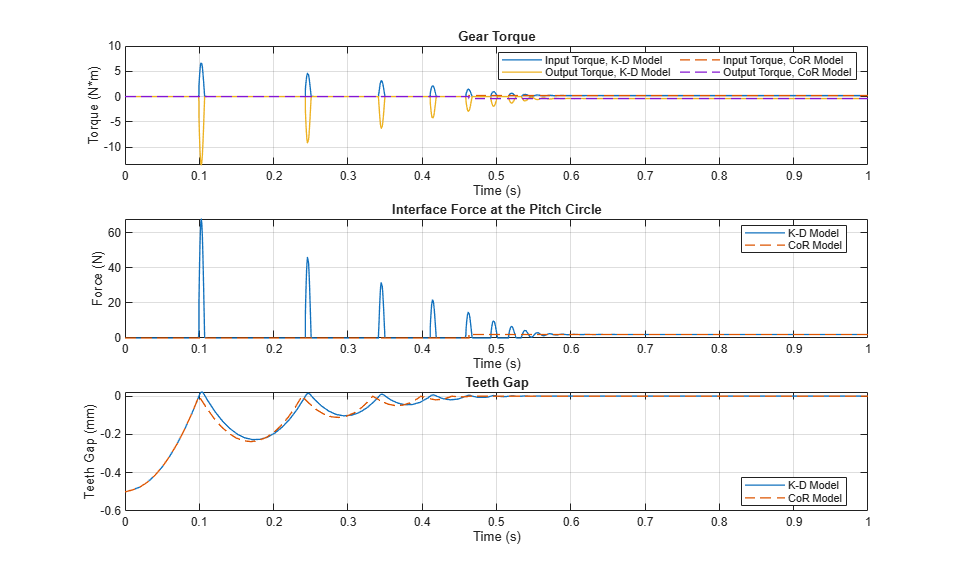# Gear with Backlash

This example shows how to model a gear with backlash using the Simple Gear block with two different backlash models: a spring-damper model and a coefficient of restitution model. The backlash is set to 1 mm, which is equivalent to 0.032 degrees rotation of the input gear that has a radius of 100 mm or 0.016 degrees rotation of the output gear that has a radius of 200 mm. Parameters in the two backlash models are adjusted so that there are a few oscillations when the torque is applied, and the models have similar behavior.

The Simscape™ Local Solver is configured so that it will provide nearly equivalent results when it is enabled. Discontinuous physical effects such as backlash require a small step size to capture the behavior accurately. The Simscape Local Solver can be enabled in the Solver Configuration block. The coefficient of restitution gear backlash model is better suited than the spring-damper backlash models for fixed step simulations such as Hardware In the Loop (HIL). In this model, the coefficient of restitution model can take step sizes ten times larger than the spring-damper model.

### Model### Simulation Results from Simscape Logging

The plot below shows the speed of two shafts connected by gears with backlash. The input shaft is driven by a constant torque. When the simulation starts, the gear is positioned in the center of the backlash gap. As the input gear strikes the output gear, each impact accelerates the output shaft. Eventually, the shafts spin at constant accelerations in response to the torque source.The plots below compare the gear torque, gear interface force, and teeth gap of the two backlash models. The model parameters have been tuned so that they have similar backlash oscilations. The Coefficient of Restitution backlash model uses instantaneous modes to simulate the impact between gears. While the instant impulses do not appear in the simscape log, the model can capture cascading dynamics in connected blocks due to the impulses. The models have identical torque behavior in steady-state when the backlash oscillations end.